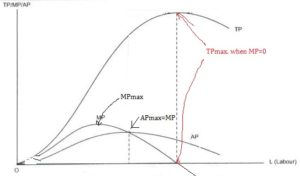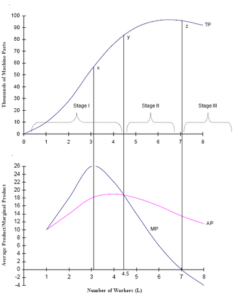# Shapes of Total Product, Average Product and Marginal Product: Concepts

The compilation of these Production and Costs Notes makes students exam preparation simpler and organised.

## Shapes of Total Product, Average Product and Marginal Product

What shapes do Total Product, Marginal product and Average Product take in the short run? Let us understand the three stages of production and the significance of each stage. Let us take a detailed look.

### Total Product

The total product refers to the total amount (or volume) of output produced with a given amount of input during a period of time. Therefore, a firm wanting to increase its Total Product in the short run will have to increase its variable factors as the fixed factors remain unchanged (that is why they are ‘fixed in the short run).

In the long run, as we know that all factors become variable, the firm can increase its total product by increasing any of its factors as all factors become variable. The concept of Total Product helps us understand what is called the Marginal Product.

### Marginal Product

The total product can be calculated by adding subsequent marginal returns to an input (also known as the marginal product). The increase in output per unit increase in input is called Marginal Product. Thus, if we were to assume Labour as the input used in the production process (say), then Marginal Product can be calculated as-

MP = Change in output/Change in input (here, labour)
TP = ƩMP

### Average Product

Average product, as the name suggests, refers to the per-unit total product of the variable factor (here, labour). Hence, the calculation of the Average Product is also very simple.

AP = Total Product/units of variable factor input = TP/L

Note that Total Product can also, therefore, be calculated as TP = AP × L

### TP, MP, AP: Shape of the Curves

When we take a look at the figure below, the following can be noted about the shapes of the TP, MP and AP curves.The TP curve first increases at an increasing rate, after which it continues to increase but at a decreasing rate, giving the curve an S-shape. This trend continues till TP reaches its maximum. Here, MP =0. After the maximum, TP starts to fall or it declines.

The MP curve also initially increases, reaches its maximum and then declines. Note that the maximum of MP is reached at the point where TP starts to increase at a diminishing rate. An interesting fact is that MP can also be negative, whereas TP is always positive even when it declines.

The AP curve also shows a similar trend as the MP. It rises, reaches its maximum, and then falls. At the point where AP reaches its maximum, AP = MP.

All – TP, MP and AP curves, are inverted U-shaped.

### Law of Variable Proportion

The law of variable proportions explains the peculiar shape of the TP curve. It is based on the following assumptions:

• Only one input is variable and all other inputs are held constant.
• The proportion in which factor units are used may be changed.
• The state of technology and factor prices are assumed to be constant.
• The time period is the short-run.

It states that if we increase one variable factor, keeping all other factors constant, the TP curve first increases at an increasing rate (convex shape) and then at a diminishing rate (concave shape) after which it starts to fall. This lends it an S-shape till the point where TP reaches its maximum.

### Stages of Production

Based on the shapes of the TP, MP, and AP curves, we can identify different stages of the production process faced by a firm.Stage I
Called the stage of increasing returns to a factor, his stage refers to that phase in the production process where MP is increasing and reaches its maximum point. It is the phase where TP is increasing at an increasing rate. The stage starts from the origin and extends till the point of inflection – the point on the TP curve after which TP increases at a diminishing rate

Since TP is increasing at an increasing rate in this phase, it is profitable for the firm to continue employing more units of the variable factor to increase its production. Hence, the firm never operates in Stage I.

Stage II
This stage is called the stage of diminishing returns to a factor. It refers to the phase where TP increases at a diminishing rate and reaches its maximum. In this phase, MP is declining but note that it still remains positive. The stage ends where MP = 0. Since this implies efficient utilization of the fixed factor, a firm always operates in the second stage of production.

Stage III
This is the final phase, called the stage of negative returns to a factor, where the TP curve starts to decline. MP in this phase becomes negative. This stage is not at all feasible for operation for any firm as the TP starts to decline, which means that production has surpassed the optimum level of specialization.

Example:

Question:
What might be possible reasons for negative returns to a factor in Stage III of production?